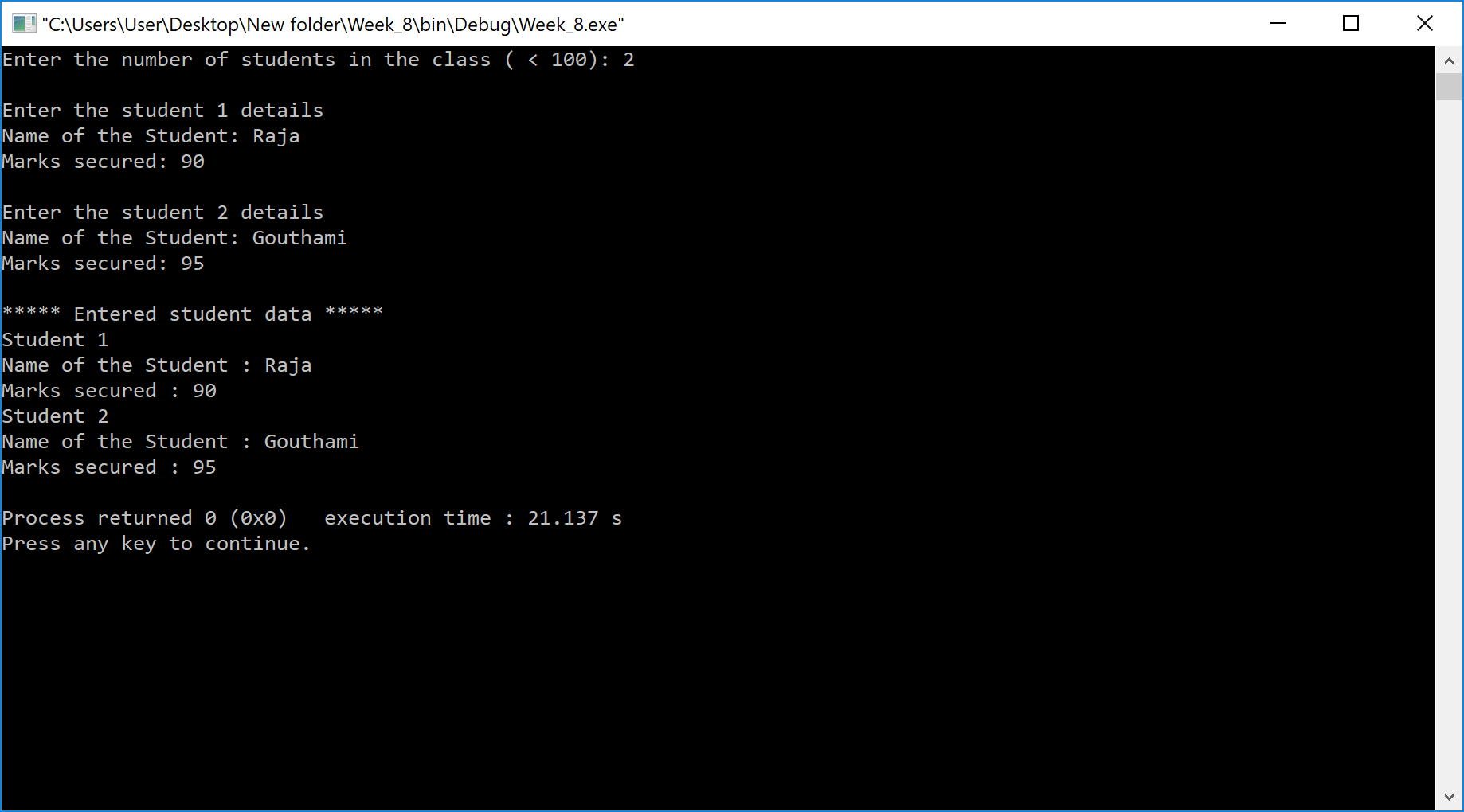The perfect place for easy learning...

×

# Aim

Write a C++ program to create an array of pointers. Invoke functions using array objects.

# Procedure

• Step 1 - Include the required header files (iostream.h and conio.h).
• Step 2 - Create a class (Student) with the following class members as public members.
• stud_name, marks as data members.
• getStudentInfo() and displayStudentInfo() as member functions.
• Step 3 - Implement the getStudentInfo() and displayStudentInfo() member functions.
• Step 4 - Create a main() method.
• Step 5 - Create an array of stud object wigth max size and a pointer object of Student class.
• Step 6 - Assign base address of object array to pointer object and make function calls to getStudentInfo() and displayStudentInfo() using class pointer object.

# Implementation

Example
``````#include <iostream>
using namespace std;

#define max 100

class Student
{
string stud_name;
int marks;
public:
void getStudentInfo(int i)
{
cout<< endl << "Enter the student " << i << " details" << endl;
cout<< "Name of the Student: ";
cin>> stud_name;
cout<< "Marks secured: ";
cin>> marks;
}
void displayStudentInfo()
{
cout << "Name of the Student : " << stud_name << endl;
cout << "Marks secured : " << marks << endl;
}
};
int main()
{
Student stud[max],*ptr;
int class_size;
ptr=stud;

cout<< "Enter the number of students in the class ( < " << max << "): ";
cin>> class_size;

for( int i=1; i<=class_size; i++ )
{
(ptr+i)->getStudentInfo(i);
}

cout<< endl << "***** Entered student data *****" << endl;

for( int i=1; i<=class_size; i++ )
{
cout << "Student " << i << endl;
(ptr+i)->displayStudentInfo();
}
return 0;
}
``````

# Result